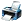# How total outcome= 25 and ekta and shyam goes to shop by 5 ways. What is this I can't understand. Please elaborate

11,114 viewsposted Jun 16, 2017

Ekta can go to shop in different 5 days from tuesday to saturday . Similarly Shyam also go to in different 5 days . There fore when one action is done in n different ways, and for each of n ways another action can be done in m ways, the total no. of actions ( or ways) is n×m . Here n=5, m=5 . So total no. of ways is 5×5=25 . Hope you understand Mr. Ajay .answer Jun 18, 2017 by
Similar Questions

When is the JEE Exams conducted.Provide me the complete information

p(x) is a polynomial of degree 5 and has extremum at -1 and 1 and lim x -0(p(x)/x^3 -2)=4 if m and M are maximum and minimum value of y=f'(x) on the set A={x|x^2+6x<5x} then m/M is

(i) Convert 14 mm to its equivalent in m.
(ii) Convert 35 kg to its equivalent in g.
(iii) Convert 57 mL to its equivalent in L.
(iv) Convert a speed of 88 m/s to its equivalent in cm/s.
(v) Convert a density of 9.45 g/L to its equivalent in g/mL.
(vi) The density of mercury metal is 1.6 g/mL. What is the mass of 3.55 mL of the metal?
(vii) The density of lead is 11.3 g/mL. What is the mass of 45 mL of the metal?
(viii) The density of table salt, NaCl, is 2.16 g/mL. what is the mass of 100.0 mL of this solid?
(ix) A particle moves through a gas at a speed of 15 km/s. How far will it move in 5.5 s?
(x) A mole of copper contains 6.02×1023 atoms. How many atoms are there in 0.525 moles?
(xi) A solution of barium nitrate contains 61.2 g per liter of solution. How many grams of barium nitrate is
contained in 2.75 L of this solution?
(xii) A sample of seawater contains 0.000245 g of sodium chloride per mL of solution. How much sodium
chloride is contained in 50.0 mL of this solution?